# 露一手，利用Python分析房價

## theme: smartblue

• 單、多變數分析
• 相關性分析
• 缺失值和異常值處理
• 啞變數轉換## 排名榜## 資料介紹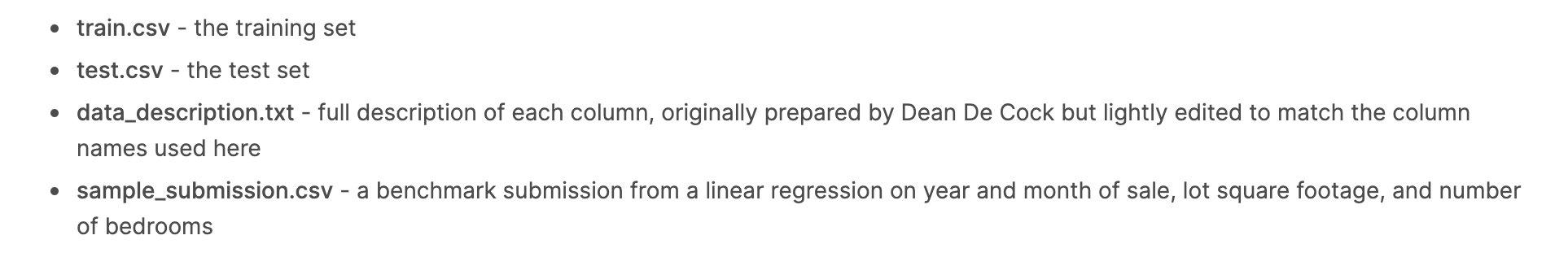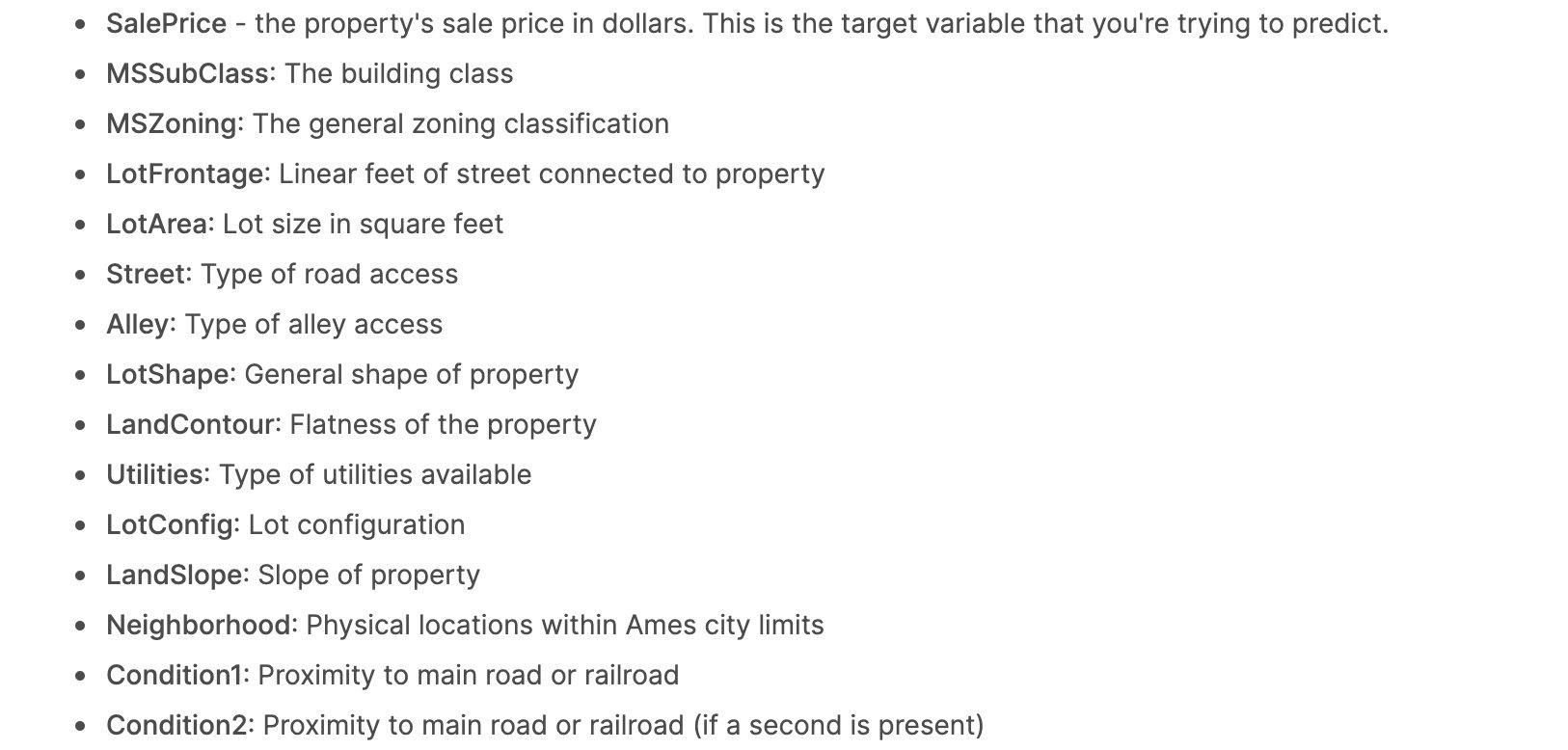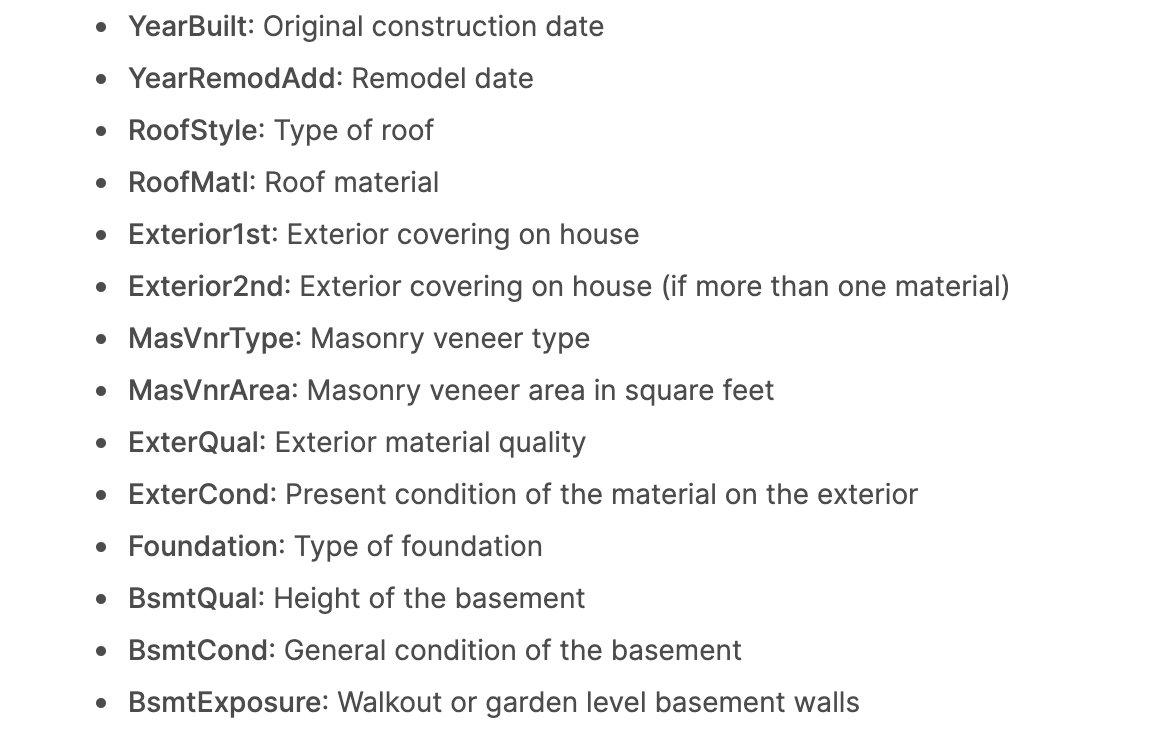## 資料EDA

### 匯入庫

```python import pandas as pd import numpy as np

# 繪圖相關

import plotly.express as px import matplotlib.pyplot as plt import seaborn as sns plt.style.use("fivethirtyeight")

# 資料建模

from scipy.stats import norm from scipy import stats from sklearn.preprocessing import StandardScaler

# 警告

import warnings warnings.filterwarnings('ignore') %matplotlib inline ```

### 匯入資料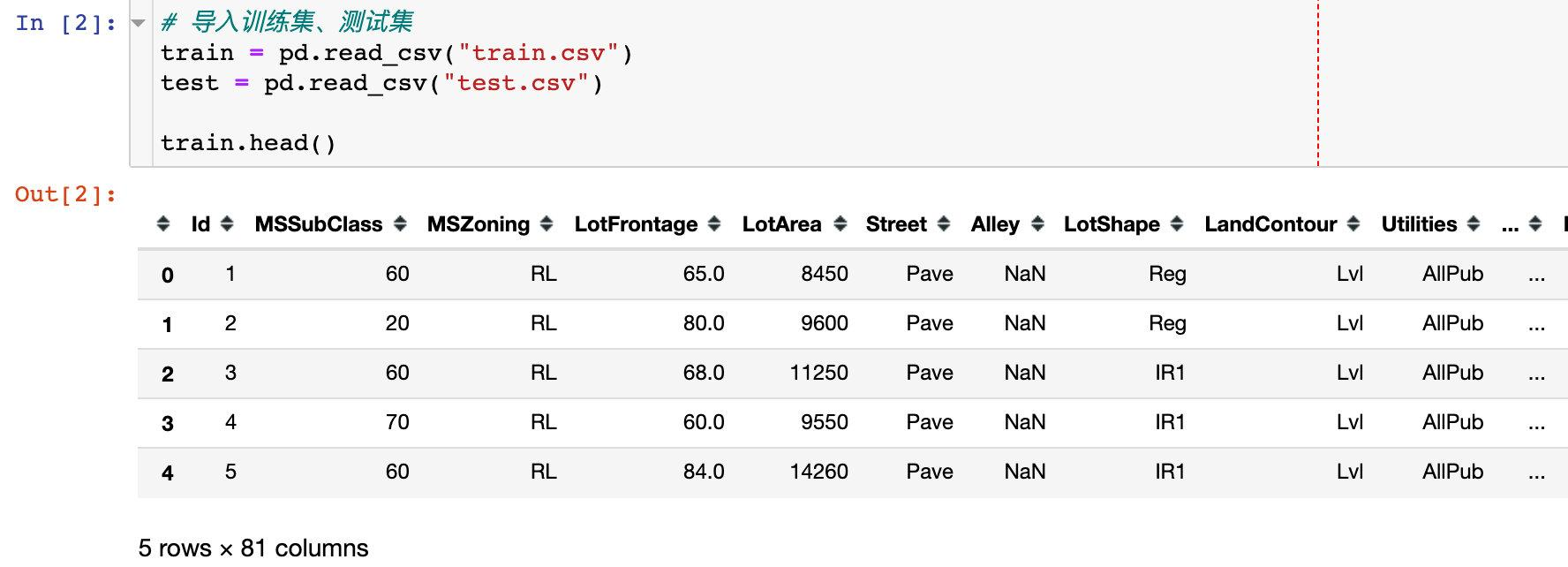### 資料資訊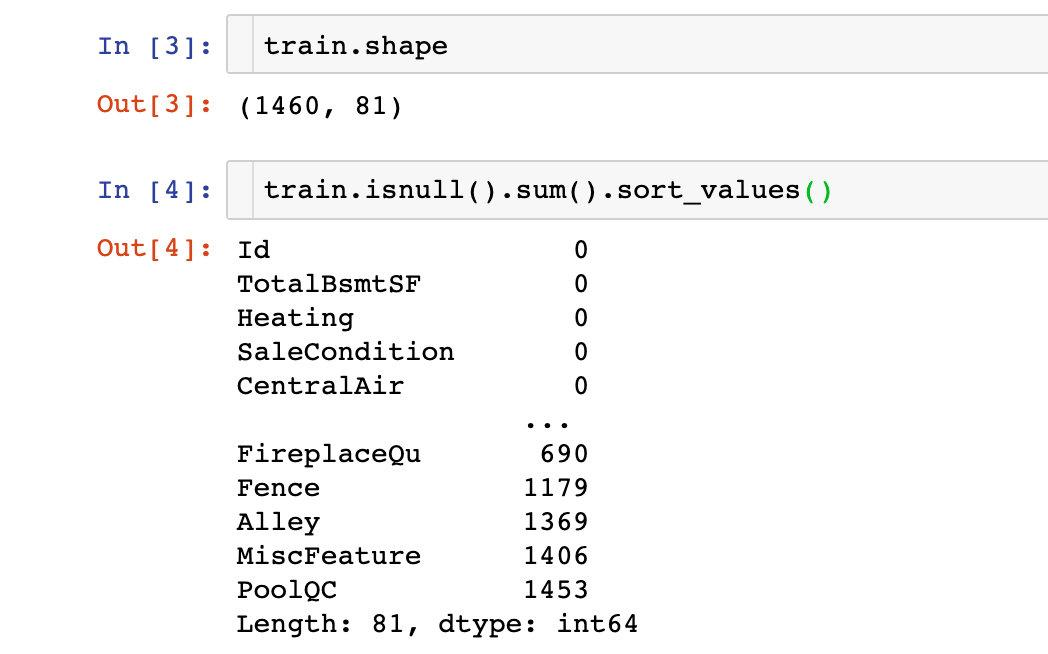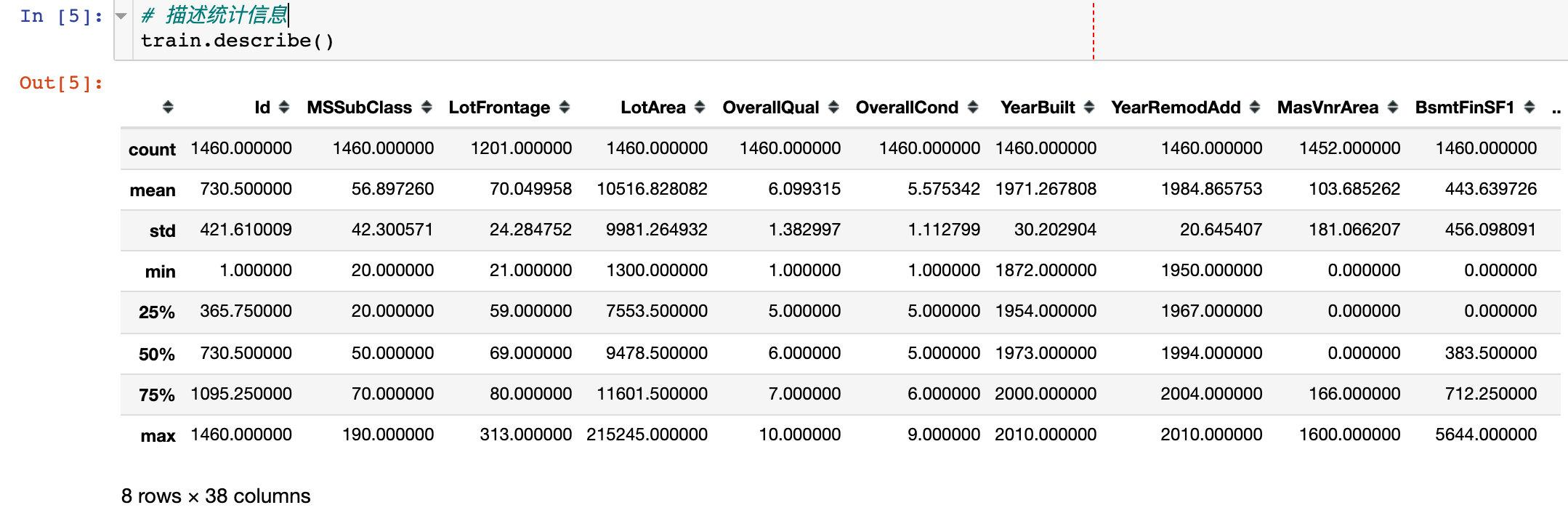## 銷售價格SalePrice分析

### 統計資訊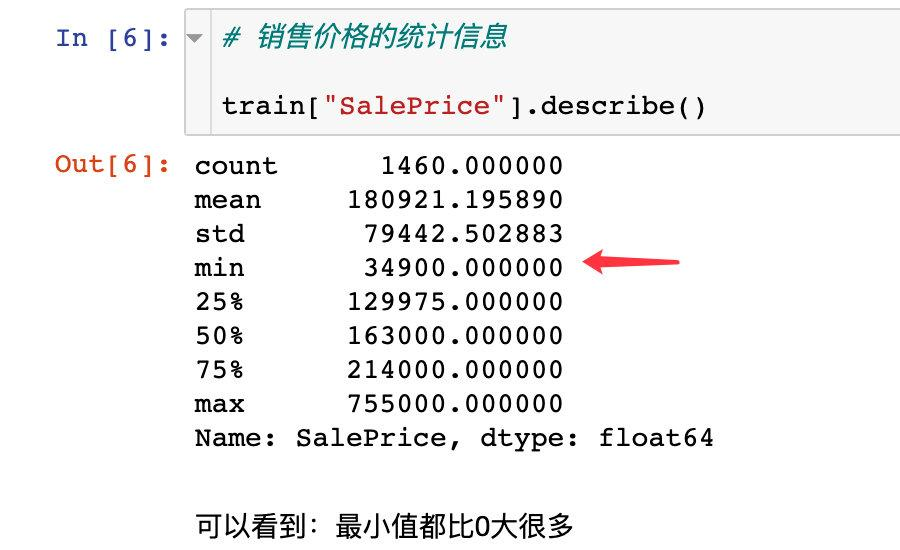• 價格的分佈偏離了正態分佈
• 有明顯的正偏度現象
• 有明顯的峰值出現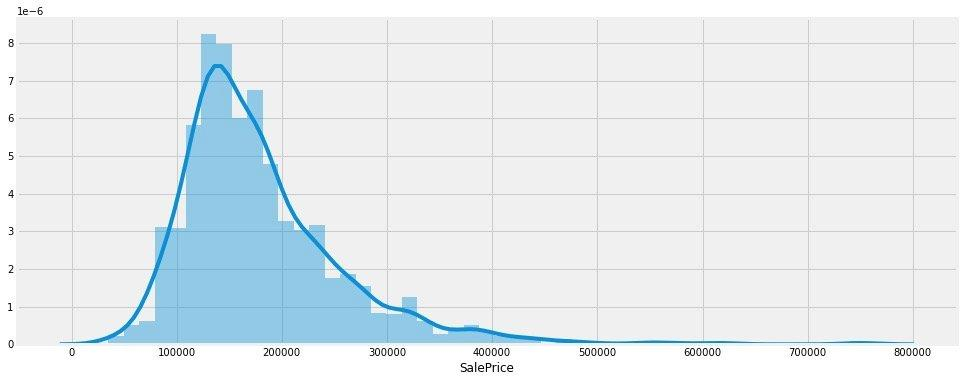### 偏度和峰度（skewness and kurtosis）

• 偏度：衡量隨機變臉概率分佈的不對稱性，是相對於平均值不對稱程度的度量，通過對偏度係數的測量，我們能夠判定資料分佈的不對稱程度以及方向。
• 峰度：是研究資料分佈陡峭或者平滑的統計量，通過對峰度係數的測量，我們能夠判定資料相對於正態分佈而言是更陡峭還是更平緩。峰度接近0，資料呈現正態分佈；峰度>0，高尖分佈；峰度<0，矮胖分佈

• 如果是左偏，則偏度小於0
• 如果是右偏，則偏度大於0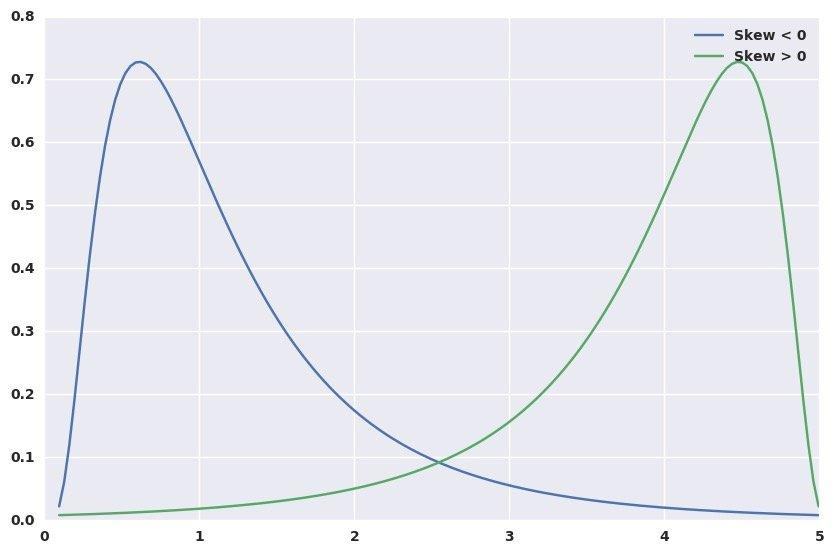• 如果是高瘦型，則峰度大於0
• 如果是矮胖型，則峰度小於0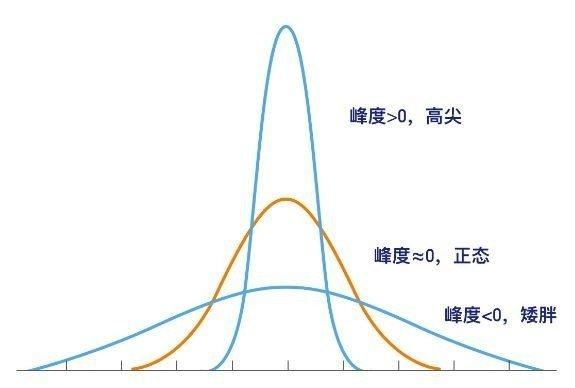```python

# 列印銷售價格的偏度和峰度

print("Skewness（偏度）: %f" % train['SalePrice'].skew()) print("Kurtosis（峰度）: %f" % train['SalePrice'].kurt())

Skewness（偏度）: 1.882876 Kurtosis（峰度）: 6.536282 ```

### SalePrice和數值型欄位的關係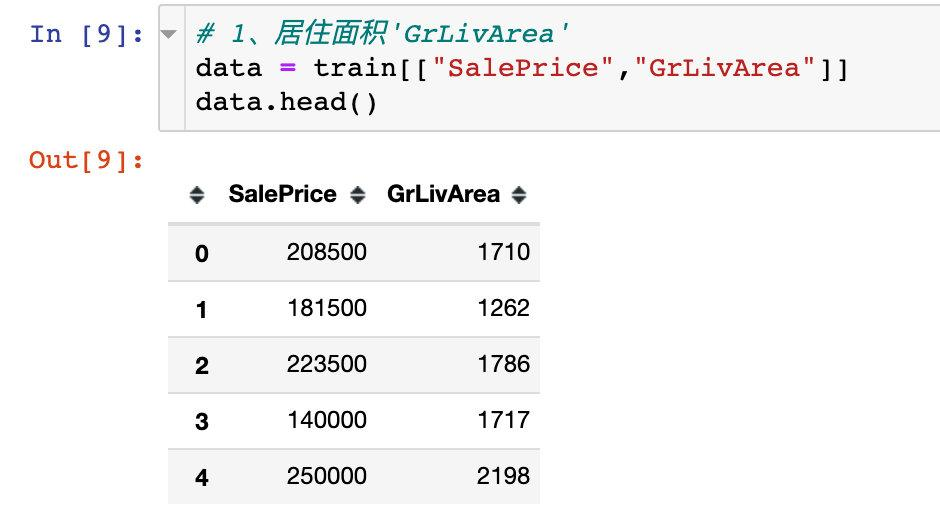```python plt.figure(1,figsize=(12,6)) sns.scatterplot(x="GrLivArea",y="SalePrice",data=data) plt.show()```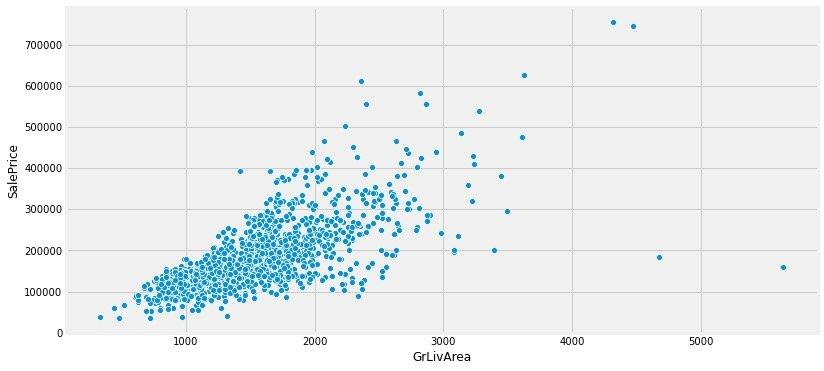```python

# plotly版本

px.scatter(data,x="GrLivArea",y="SalePrice",trendline="ols") ```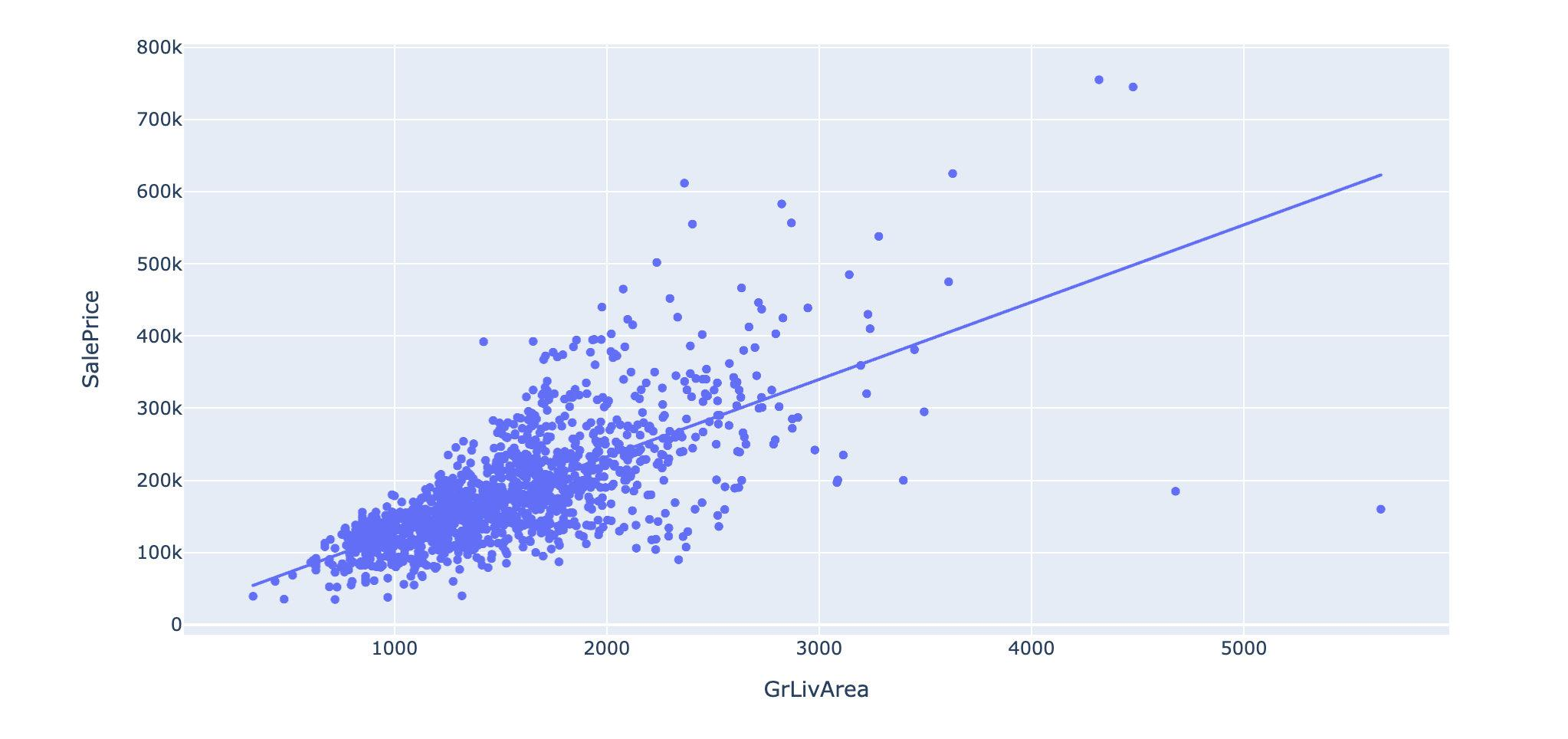```python

# 2、TotalBsmtSF

data = train[["SalePrice","TotalBsmtSF"]]

plt.figure(1,figsize=(12,6)) sns.scatterplot(x="TotalBsmtSF",y="SalePrice",data=data) plt.show() ```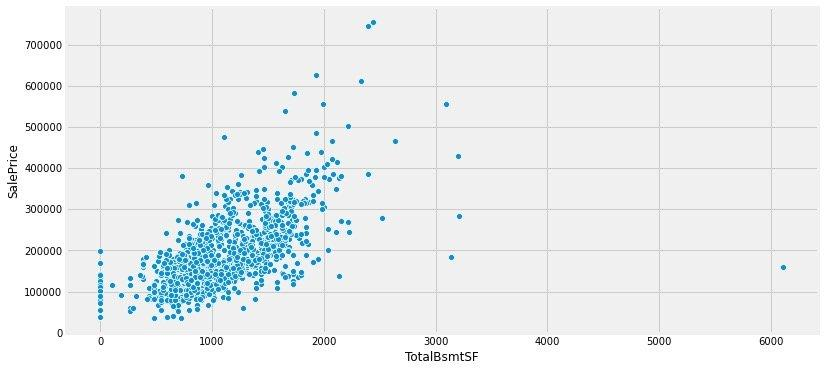### 價格和分型別欄位的關係

1、OverallQual VS SalePrice

```python

# 總共10個類別

train["OverallQual"].value_counts() ```

```python 5 397 6 374 7 319 8 168 4 116 9 43 3 20 10 18 2 3 1 2 Name: OverallQual, dtype: int64```

```python data = train[["SalePrice","OverallQual"]]

# 繪製子圖：1號位

f,ax = plt.subplots(1,figsize=(12,6)) fig = sns.boxplot(x="OverallQual",y="SalePrice",data=data)

# y軸的刻度範圍

fig.axis(ymin=0,ymax=800000) plt.show() ```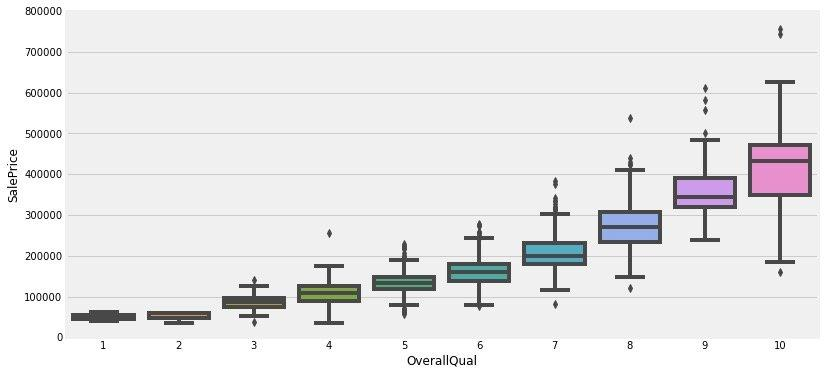2、YearBuilt VS SalePrice

```python data = train[["SalePrice","YearBuilt"]]

# 建造年份和房價的關係

f,ax = plt.subplots(1,figsize=(16,8)) fig = sns.boxplot(x="YearBuilt",y="SalePrice",data=data)

# y軸的刻度範圍

fig.axis(ymin=0,ymax=800000) plt.show() ```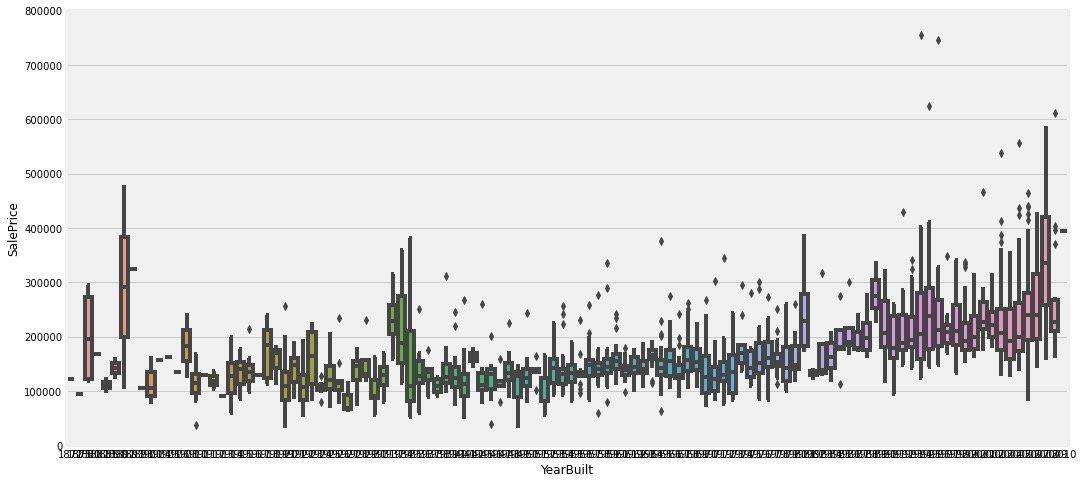### 小結

1. 地面生活區(GrLivArea)、地下室面積(GrLivArea)和銷售價格SalePrice都是呈現正向的線性相關
2. 房屋的整體質量(OverallQual)和建造年份(YearBuilt)好像也和銷售價格線性相關。常識來說，整體的質量越好，價格越貴

## 相關性分析

• 兩兩屬性間的相關性（熱力圖）
• 銷售價格saleprice和其他屬性的關係（熱力圖）
• 關聯性最大的屬性間的關係（散點圖）

### 整體相關性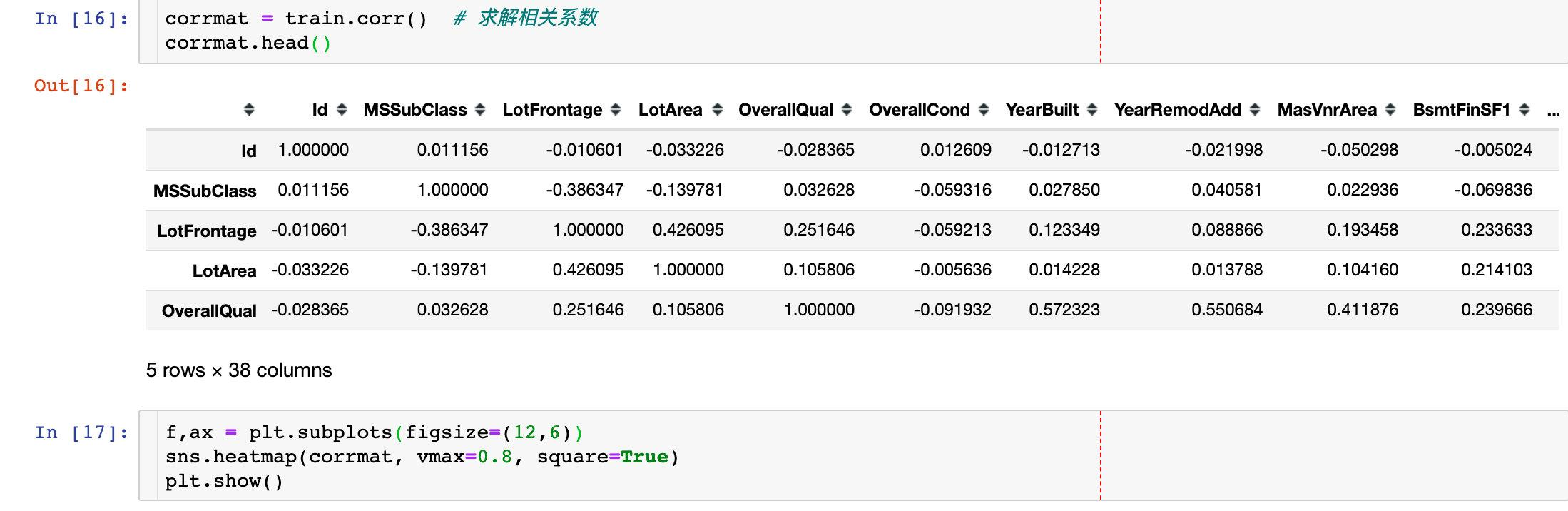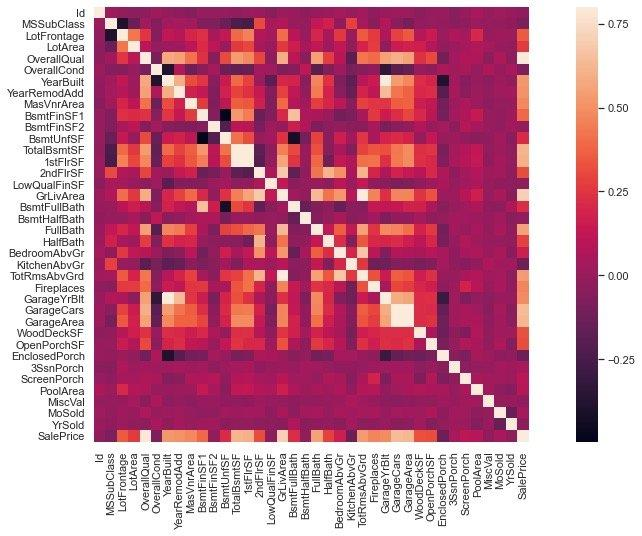• TotalBsmtSF and 1stFlrSF
• GarageCar and GarageArea

### 縮放相關矩陣（銷售價格saleprice）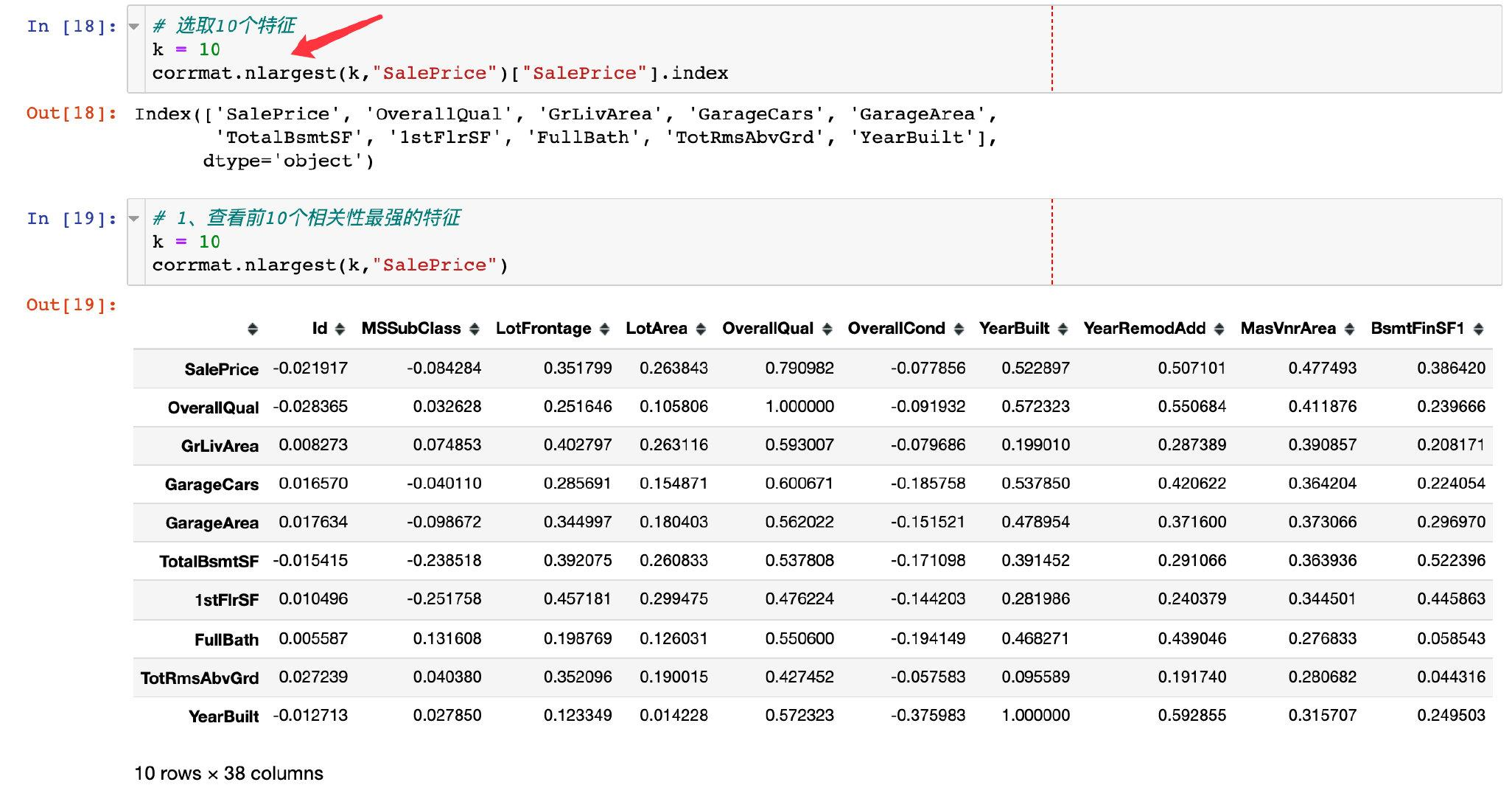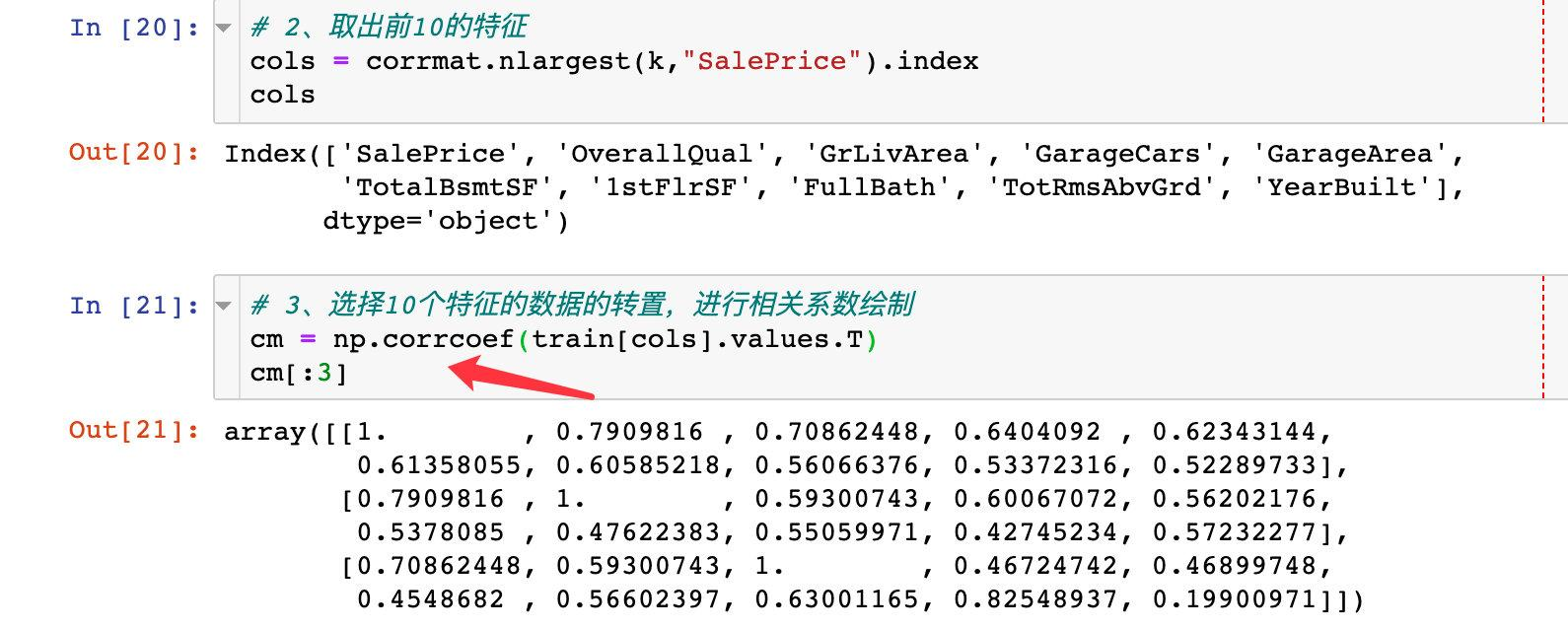```python sns.set(font_scale=1.25) hm = sns.heatmap( cm, # 繪圖資料 cbar=True, # 是否將顏色條作為圖例，預設True annot=True, # 是否顯示數值 square=True, # 是否使熱力圖每個單元為正方形，預設為False fmt='.2f', # 保留兩位小數 annot_kws={'size':10}, xticklabels=cols.values, # xy軸設定 yticklabels=cols.values)

plt.show() ```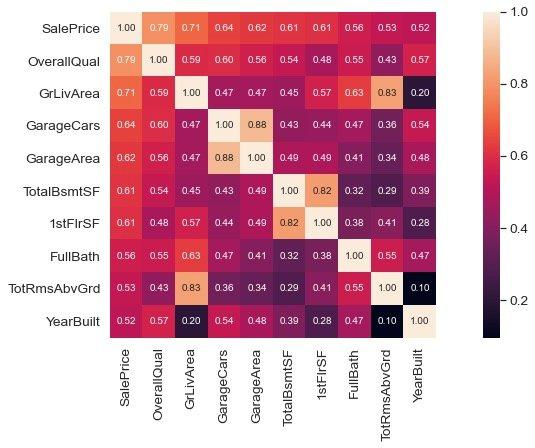### 小結1

• 'OverallQual', 'GrLivArea' and 'TotalBsmtSF'是真的和'SalePrice'呈現強相關
• 'GarageCars' and 'GarageArea' 也是兩個相關性比較強的特徵；而且他們都是同時出現，後續選取GarageCars進行分析
• 建築年限'YearBuilt'相對來說，相關性比較低

### 變數離散圖

```python sns.set()

# 待分析的變數

cols = ['SalePrice', 'OverallQual', 'GrLivArea', 'GarageCars', 'TotalBsmtSF', 'FullBath', 'YearBuilt'] sns.pairplot(train[cols],size=2.5) plt.show() ```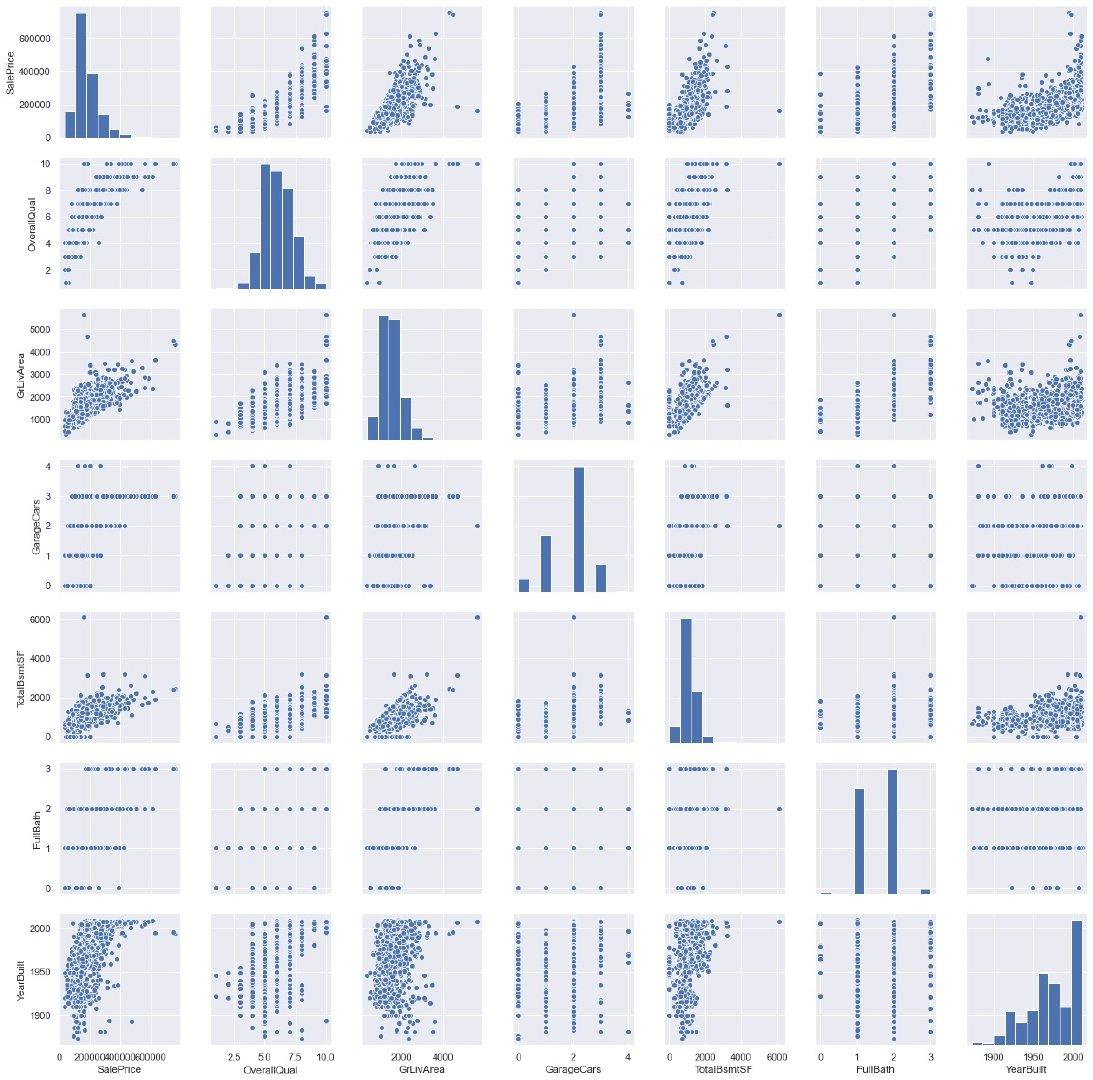## 缺失值處理

• 缺失值分佈情況怎麼樣？
• 缺失值是隨機的？還有具有某種規律

1、檢視每個欄位的缺失值情況

```python

# 每個欄位的缺失值數量：降序

```python PoolQC 1453 MiscFeature 1406 Alley 1369 Fence 1179 FireplaceQu 690 dtype: int64```

2、轉成百分比

```python

# 每個欄位的缺失值 / 總數

percent = (train.isnull().sum() / train.isnull().count()).sort_values(ascending=False) percent.head() ```

```python PoolQC 0.995205 MiscFeature 0.963014 Alley 0.937671 Fence 0.807534 FireplaceQu 0.472603 dtype: float64```

3、資料合併，整體的缺失值情況：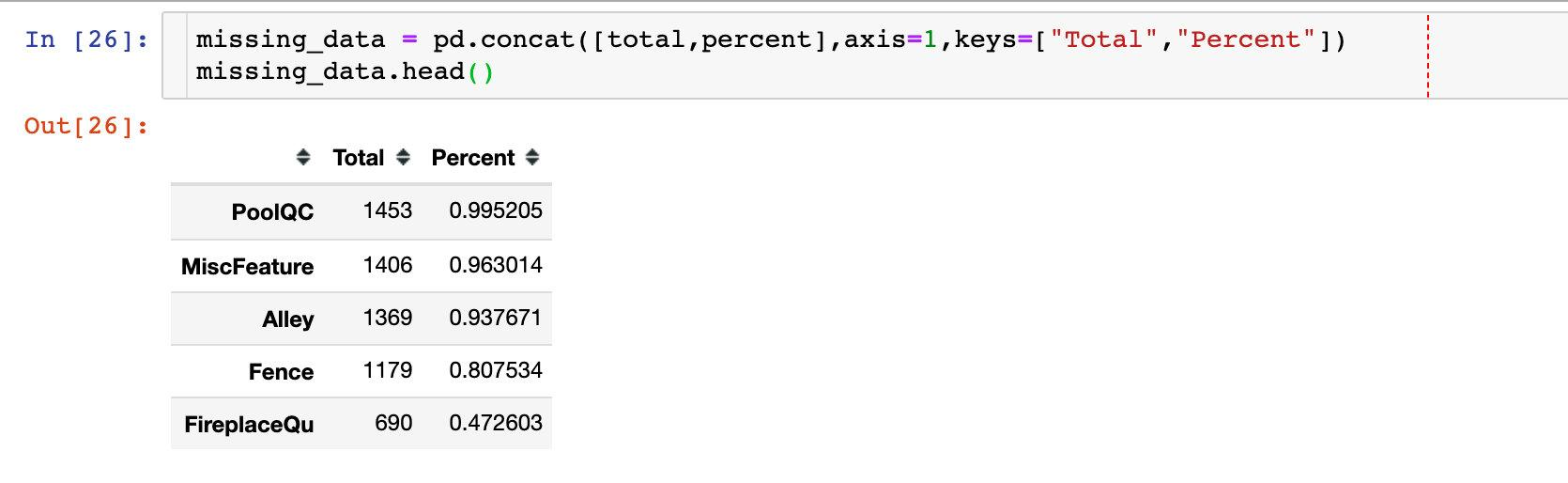### 刪除缺失值

In summary, to handle missing data,

1、we'll delete all the variables with missing data, except the variable 'Electrical'.

2、In 'Electrical' we'll just delete the observation with missing data.

```python

# 步驟1：需要刪除的欄位

missing_data[missing_data["Total"] > 1].index ```

```python Index(['PoolQC', 'MiscFeature', 'Alley', 'Fence', 'FireplaceQu', 'LotFrontage', 'GarageYrBlt', 'GarageCond', 'GarageType', 'GarageFinish', 'GarageQual', 'BsmtFinType2', 'BsmtExposure', 'BsmtQual', 'BsmtCond', 'BsmtFinType1', 'MasVnrArea', 'MasVnrType'], dtype='object')```

```python

# 第一步

train = train.drop(missing_data[missing_data["Total"] > 1].index,1)

# 第二步

train = train.drop(train.loc[train["Electrical"].isnull()].index) ```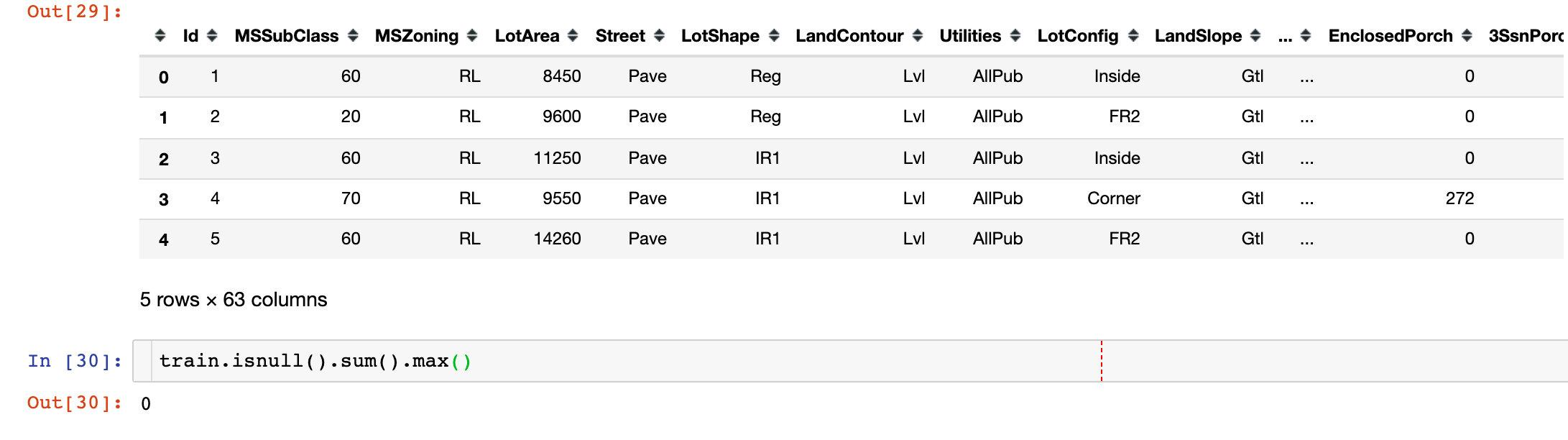```python

# np.newaxis 增加資料維度，一維變成二維

saleprice_scaled = StandardScaler().fit_transform(train["SalePrice"][:,np.newaxis]) saleprice_scaled[:5] ```

```python array([[ 0.34704187], [ 0.0071701 ], [ 0.53585953], [-0.5152254 ], [ 0.86943738]])```

```python

# argsort：返回的是索引值；預設是升序排列，最小的在最前面，最大的在最後

low_range = saleprice_scaled[saleprice_scaled[:,0].argsort()][:10]

high_range = saleprice_scaled[saleprice_scaled[:,0].argsort()][-10:]

print(low_range) print('----------') print(high_range) ```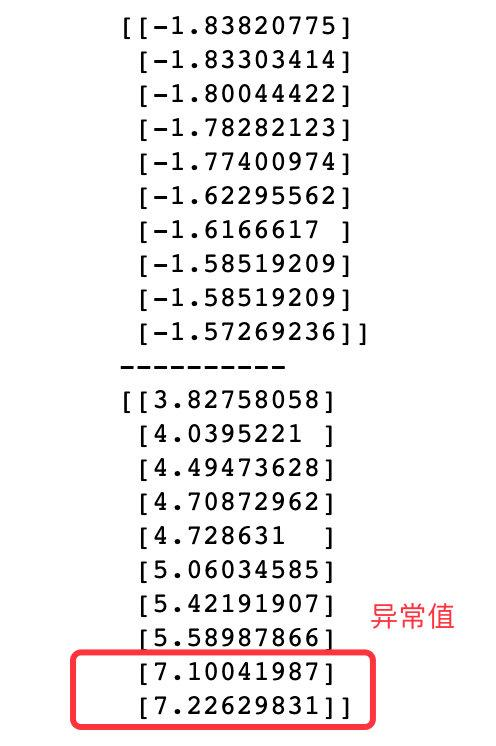### 小結3

• low_range接近，且離0比較近
• high_range離0很遠；且7+的資料就應該是離群點了

### 單變數分析1

```python data = train[["SalePrice","GrLivArea"]] data.plot.scatter(x="GrLivArea",y="SalePrice",ylim=(0,800000)) plt.show()```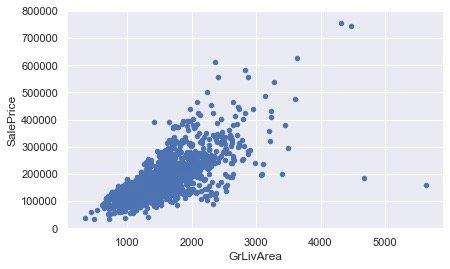### 刪除離群點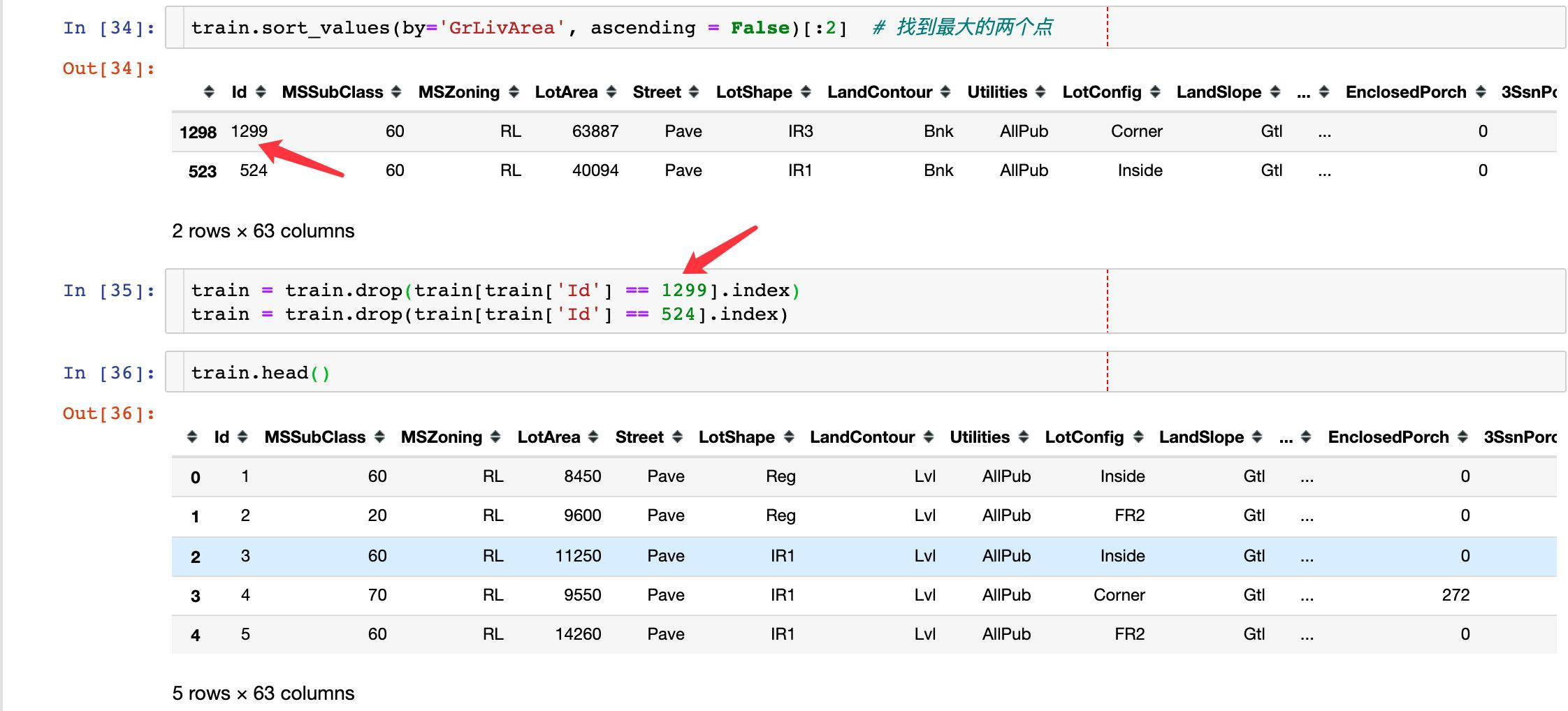### 單變數分析2

```python data = train[["SalePrice","TotalBsmtSF"]] # 待分析的兩個變數 data.plot.scatter(x="TotalBsmtSF",y="SalePrice",ylim=(0,800000)) plt.show()```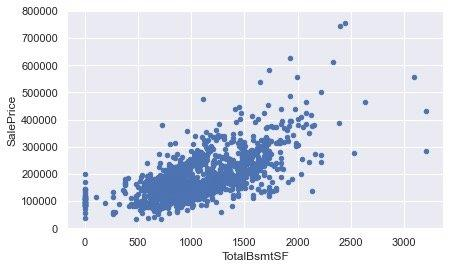## 深入理解SalePrice

• Normality：歸一化
• Homoscedasticity：同方差性
• Linearity：線性特質
• Absence of correlated errors：相關誤差

### Normality歸一化（SalePrice）

```python sns.distplot(train["SalePrice"],fit=norm) fig = plt.figure() res = stats.probplot(train["SalePrice"], plot=plt)```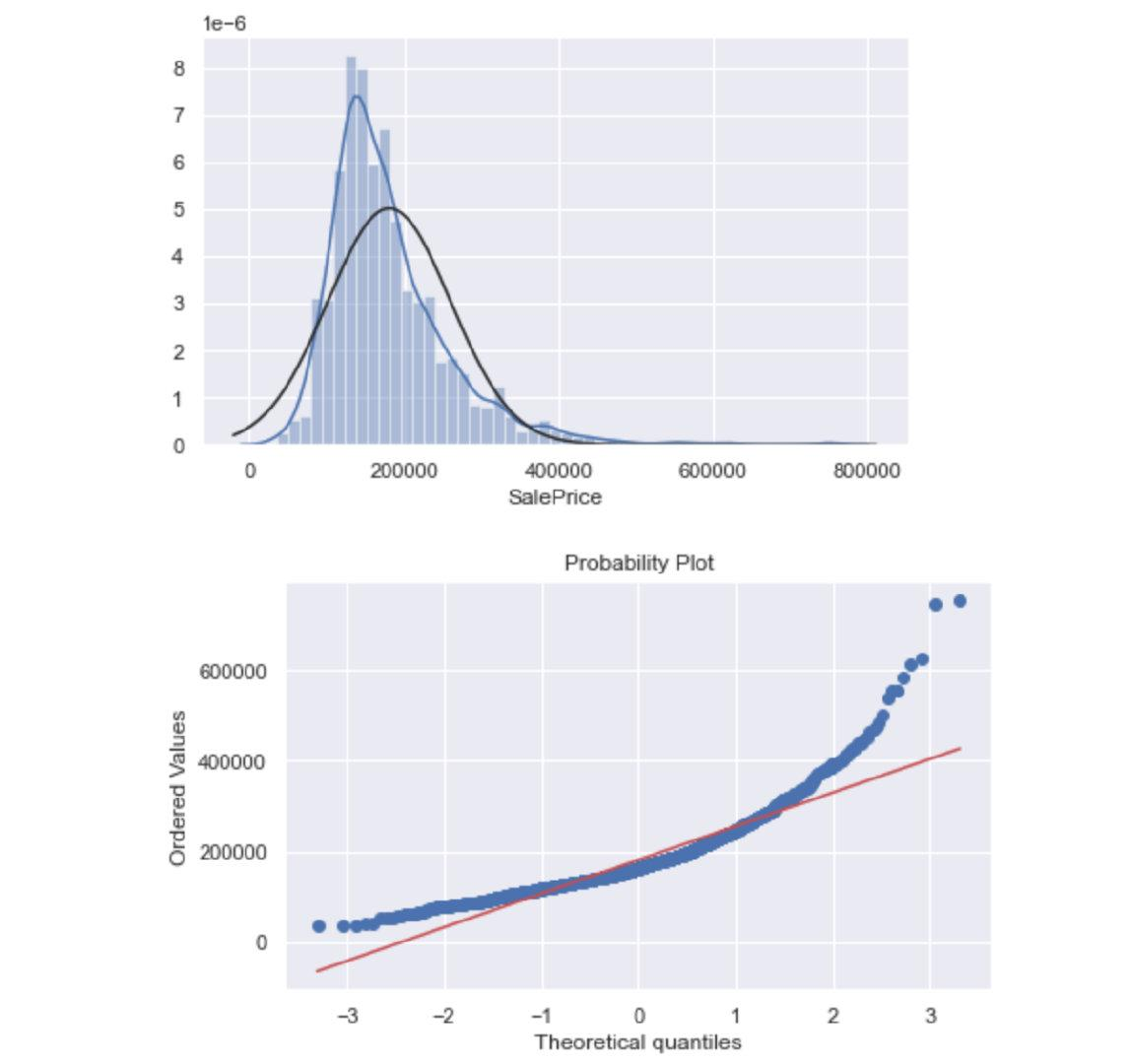```python

## 對數變換

train["SalePrice"] = np.log(train["SalePrice"])

sns.distplot(train["SalePrice"],fit=norm) fig = plt.figure() res = stats.probplot(train["SalePrice"], plot=plt) ```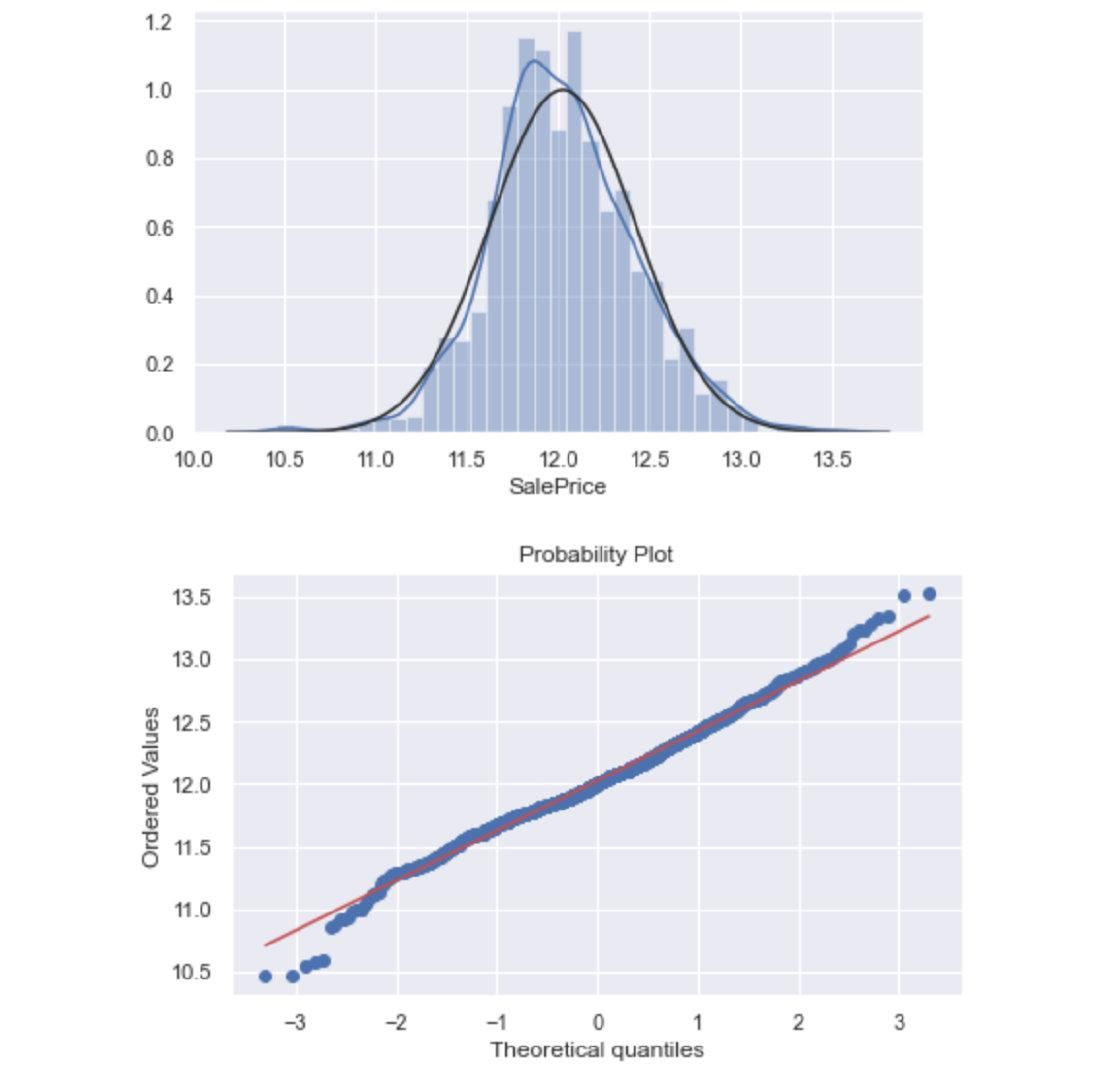### Normality-歸一化（GrLivArea）

```python sns.distplot(train["GrLivArea"],fit=norm) fig = plt.figure() res = stats.probplot(train["GrLivArea"], plot=plt)``````python

# 執行相同的對數操作

train["GrLivArea"] = np.log(train["GrLivArea"])

sns.distplot(train["GrLivArea"],fit=norm) fig = plt.figure() res = stats.probplot(train["GrLivArea"], plot=plt) ```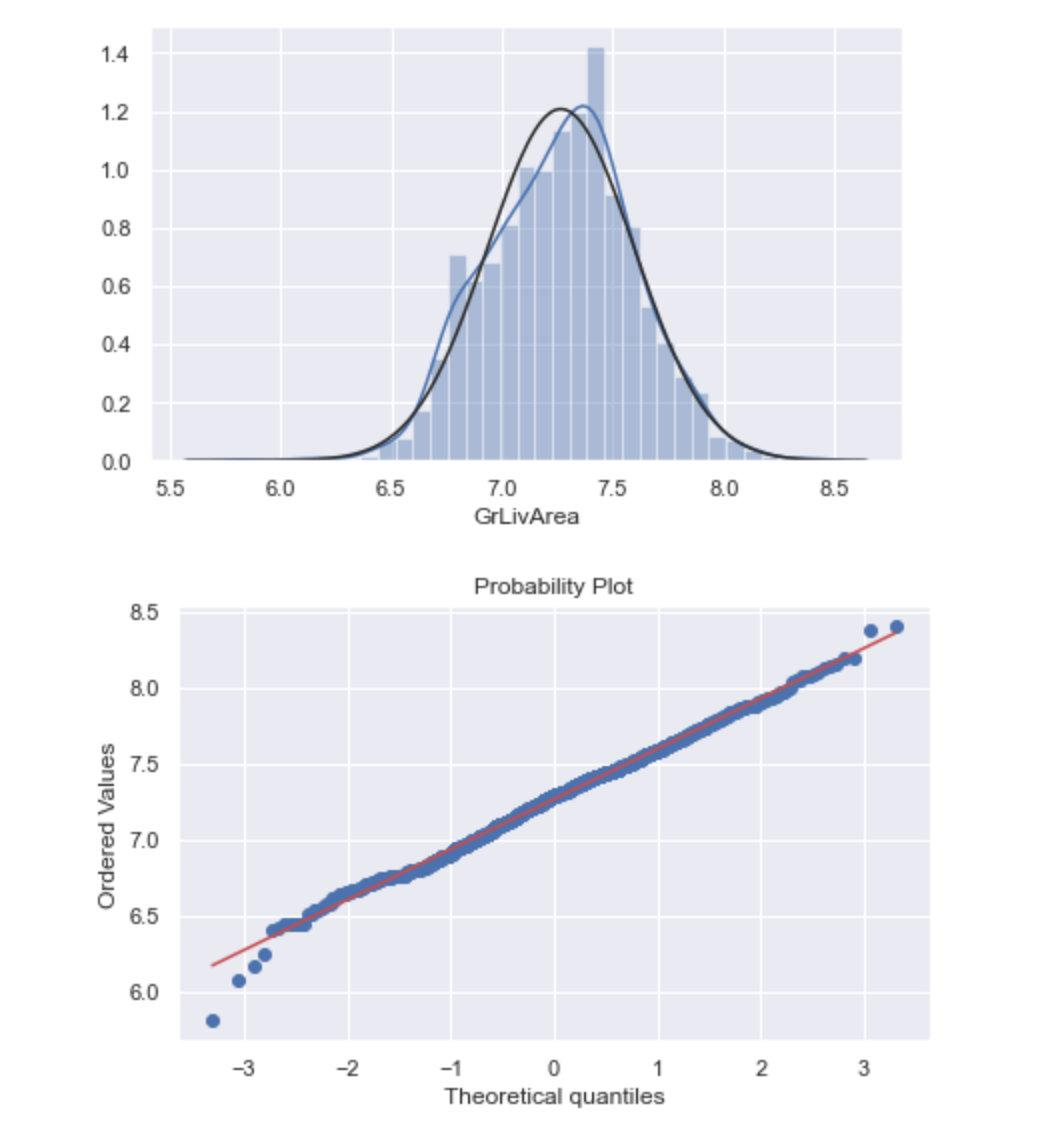### Normality-歸一化（TotalBsmtSF）

```python sns.distplot(train["TotalBsmtSF"],fit=norm) fig = plt.figure() res = stats.probplot(train["TotalBsmtSF"], plot=plt)```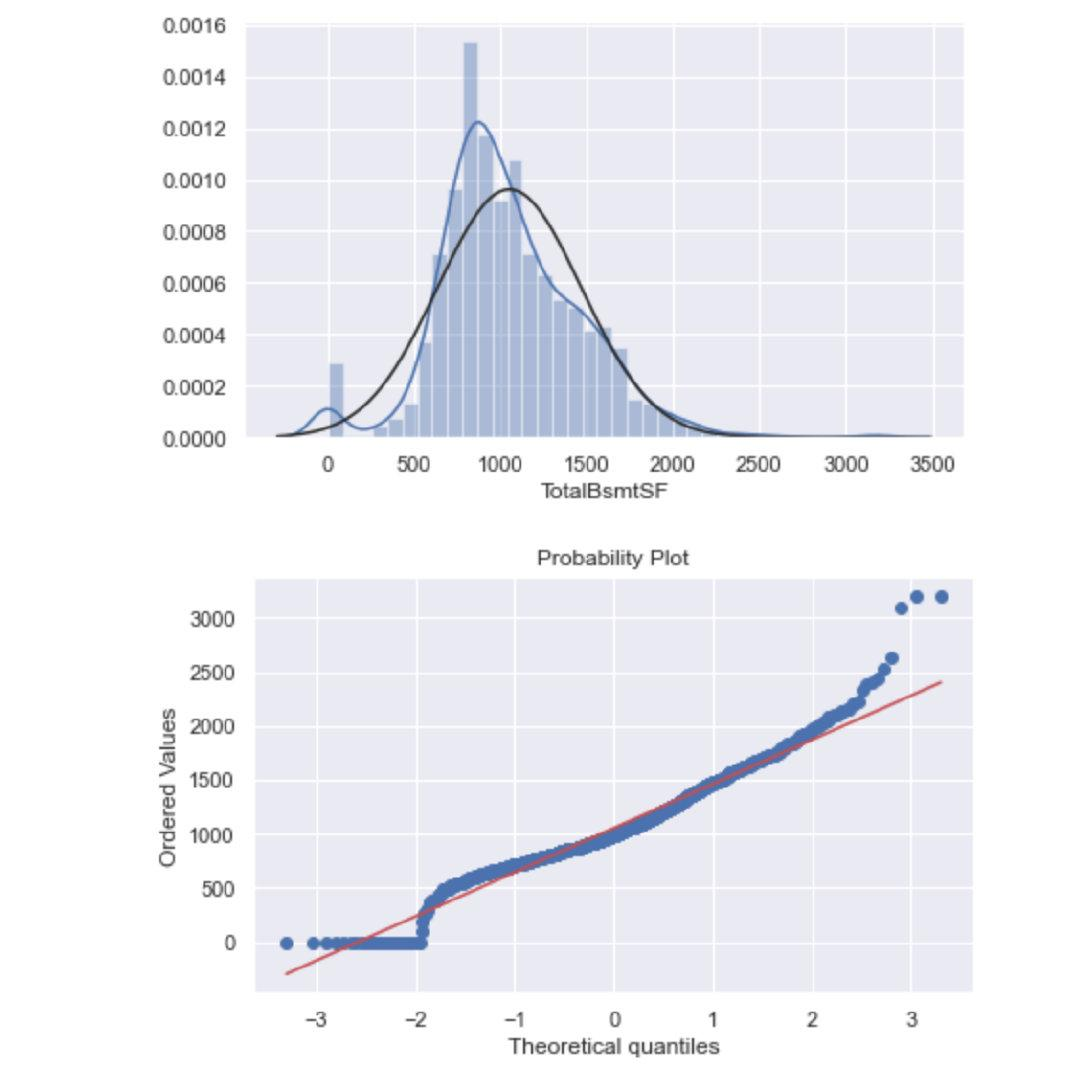```python

# 增加一列資料

train['HasBsmt'] = 0

# 當TotalBsmtSF>0 則賦值1

train.loc[train['TotalBsmtSF']>0,'HasBsmt'] = 1

# 對數轉換：等於1的部分

train.loc[train['HasBsmt']==1,'TotalBsmtSF'] = np.log(train['TotalBsmtSF'])

# 繪圖

data = train[train['TotalBsmtSF']>0]['TotalBsmtSF'] sns.distplot(data,fit=norm) fig = plt.figure() res = stats.probplot(data, plot=plt) ```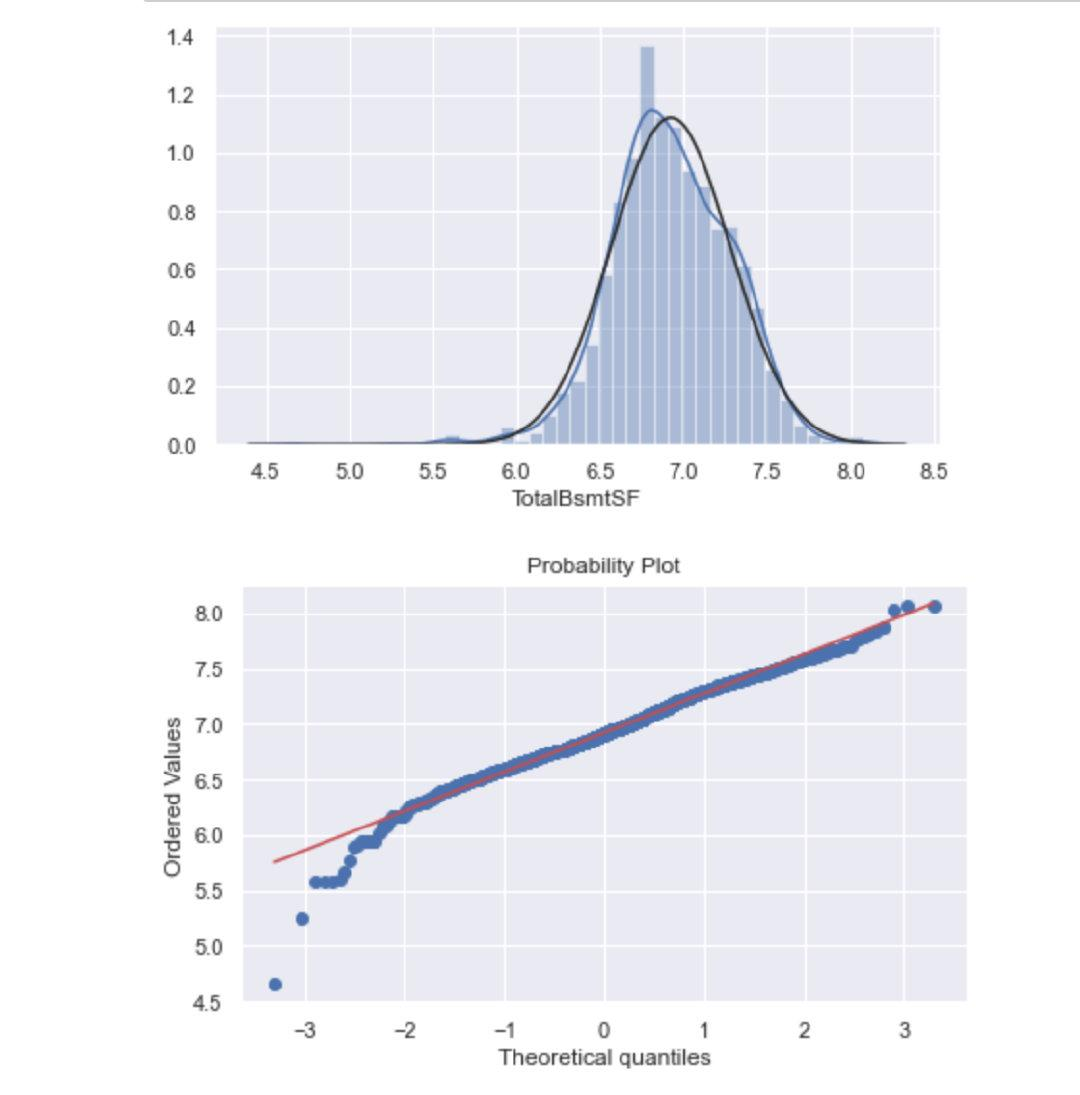## 同方差性

The best approach to test homoscedasticity for two metric variables is graphically

1、討論：'SalePrice' 和'GrLivArea'之間的關係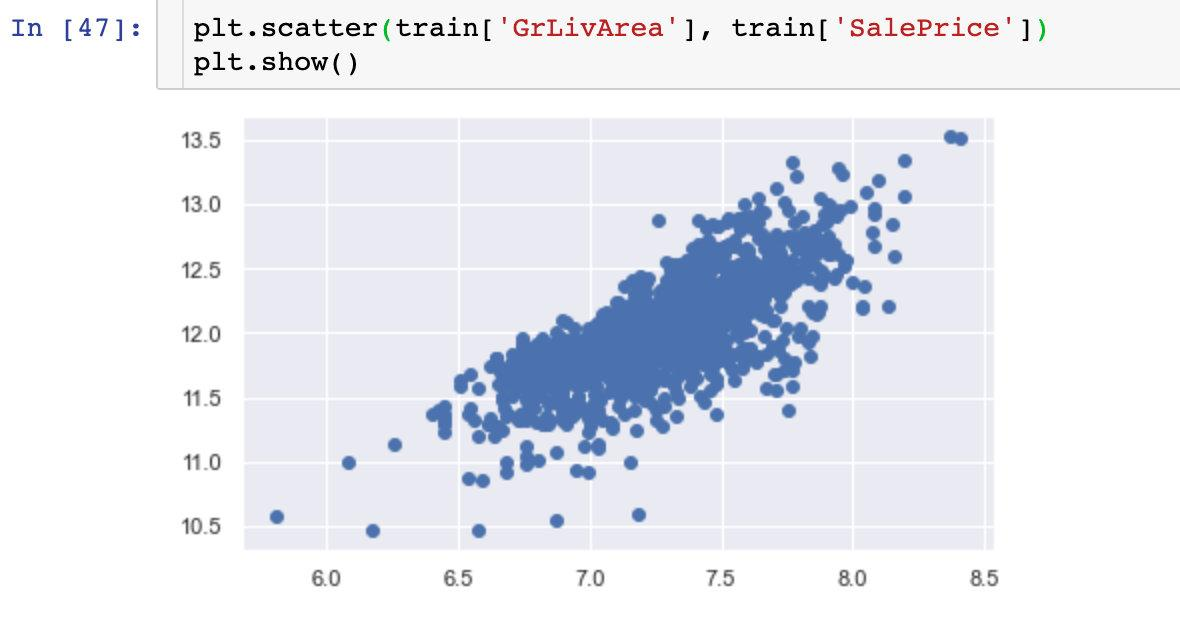2、討論SalePrice' 和 'TotalBsmtSF'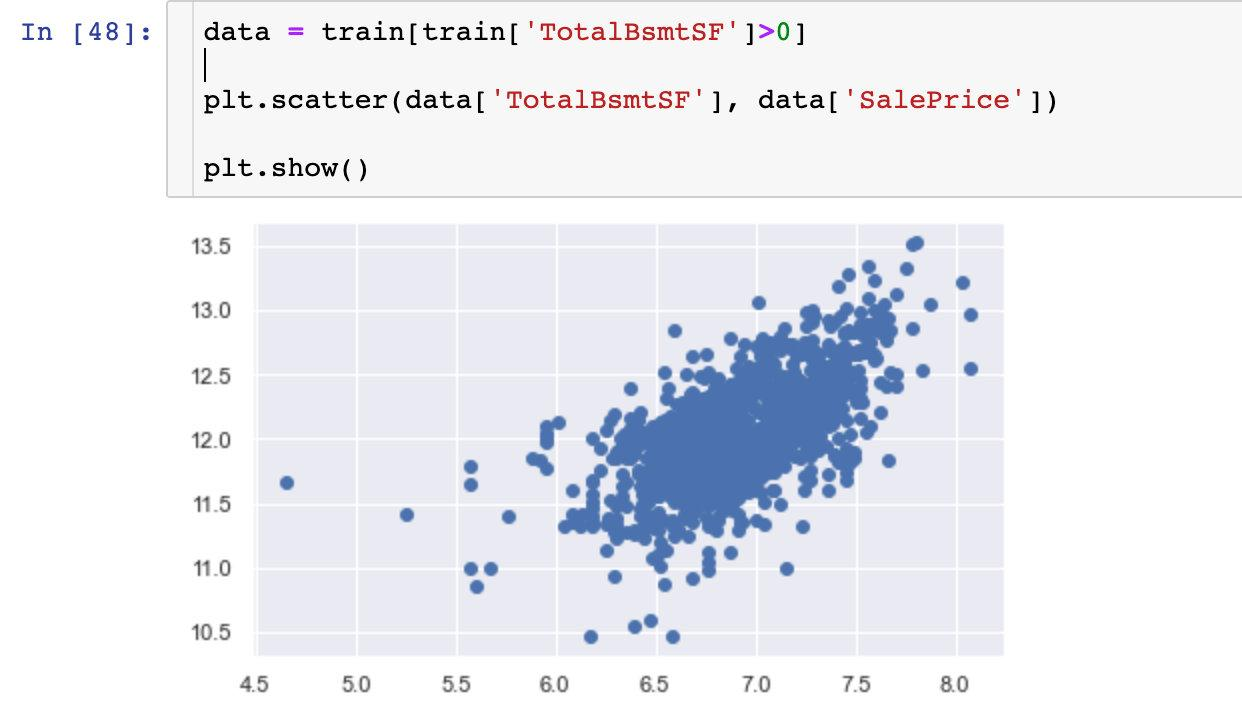We can say that, in general, 'SalePrice' exhibit equal levels of variance across the range of 'TotalBsmtSF'. Cool!

## 生成啞變數

Pandas中的get_dummies函式能夠實現：

```python train = pd.get_dummies(train) # 生成啞變數 train```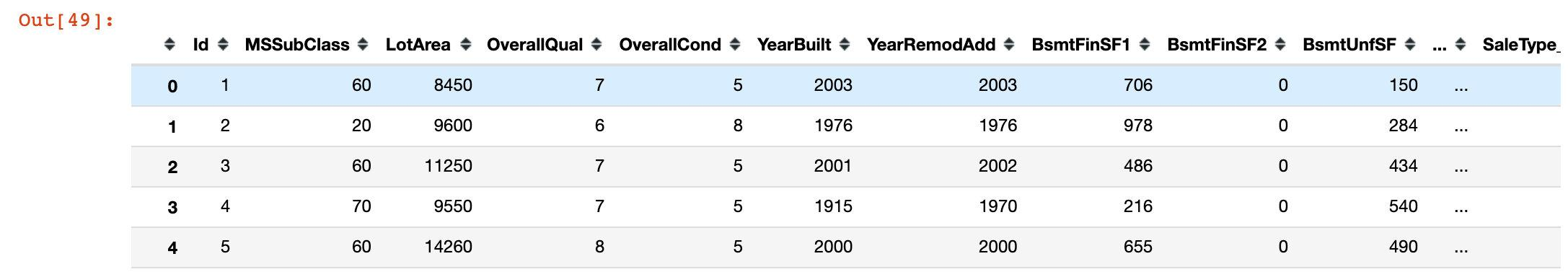## 總結

1. 整體變數間的相關性分析
2. 重點分析了變數“SalePrice”
3. 處理缺失值和異常值（離群點）
4. 做了一些統計分析，將分類變數變成了啞變數

• 多元統計分析
• 偏度和峰度
• 啞變數的深入
• 標準化和歸一化

「其他文章」